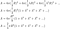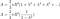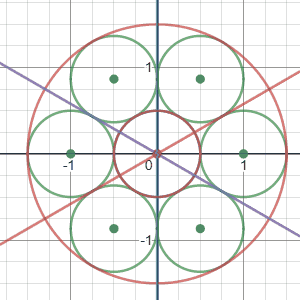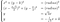# Jane Street June ’20 (Circle Time)

Well, well, well, jane street is back at it again with another puzzle this month, and today might be the last day I can solve it before the official solution is posted, so let’s get to it!

Let’s make a diagram of the problem to visualize a “ring” of circles. I’m using desmos, but feel free to use other software like geogebra, etc.

To maximize the area of the ring of circles, we’ll place the center of the “ring” at the center of circle C and make the ring large enough such that every circle touches circle C exactly once.

From the diagram, we can see that the ring of circles comprises an area of

where r is the radius of each green circle or a third of the radius of the circle C. The original circle’s area can be written as:

Where R is the radius of the circle C. The proportion is then:

But we’re not done here — all we know now is that the proportion will be greater than at least 66.6%.

We can place another, smaller “ring” with its center at the center of the circle C. The radius of the circles of this smaller “ring” should be scaled down by a constant factor λ. Since the next ring of circles will look just like the largest ring, we can place another ring of circles with each circle’s radius scaled down by another λ, and another, and so on to place an infinite number of rings of circles.

But how do we sum the area of an infinite ring of circles? With a geometric series! If you’ve forgotten about geometric series, don’t worry! I just wrote my AP Calculus BC exams, so it’s still fresh in my mind. A geometric series is a series with a constant ratio between terms. A geometric series could be 1,2,4,8,16, and so on since the ratio between consecutive terms is 2. Our geometric series will be composed of the area of each ring of circles.The sum of the geometric series of the rings of circles

This doesn’t really look like a geometric series, so let’s wrestle it into something more manageable.The geometric series of λ² can be seen with a coefficient

We know that our geometric series will sum to a value (known as a “convergent” geometric series) since each circle of a ring must have a smaller radius than the previous ring of circles.

For our convergent geometric series, the sum is defined as:

Where a is the first area of the “ring” in the series, and r is the common ratio between areas of “rings”.

The common ratio between areas of “rings” will be given by λ², so our equation for the sum of the areas of “rings” can be expressed as:

We can substitute this back into our original sum.Notice that we’ve replaced the infinite series part with a closed-form formula

We can also create an expression for the proportion now.

Now our task is to find λ, the ratio of the radii between circles of consecutive rings.

Let’s start with analytical geometry. We want to set up two circles of the next ring that touch the circles of the largest ring at exactly two points. Let’s choose k, the y-coordinate of the center of one of a blue circle, and define another, red circle in terms of k.Both blue and red circles depend solely on the chosen k

Fair warning: This approach requires an IMMENSE amount of algebraic manipulation that you won’t see here — if you’re a nerd like me and want to see the algebra, pour through the sidebar of the graph (final expressions) or the notes(derivations). Let me know if you find anything interesting!

The blue circle’s center is at (0,k), and the red circle’s center lies at the intersection between the purple line (a line that is a 60° rotation from x = 0) and the red line, (a line from the intersection between the East and North-East circles and the intersection between the West and South-West circles).

The maximum area of the ring of circles occurs when the blue and red circles touch at exactly one point. From the animation, we see that there exists a value k where this occurs. If we find this value k, we can find the radius of the blue circle, calculate the ratio between the radii of the green and blue circles, and we will have λ.

While we have equations for both blue and red circles, we have three unknowns (x,y,k) and two equations, which means that we need more equations (the more, the merrier here). From our diagram, we can make a very educated guess that the point at which the circles touch is given by the intersection between the black and purple lines.

The black line is defined as the line between the centers of the North-East circle and the South-West circle. The purple line is defined as the line between the centers of the blue and red circles.

Now we have 4 equations and three unknowns — more than we need to solve for k.Our awesome system of equations that will let us solve for k

Using the last two equations, we can create an expression for the x and y coordinates of the intersection!

Using those values and the other equations we get a QUARTIC equation for k, of which we want only the first real solution.yikes…I don’t want to solve this… Oh, wait… I’m writing the article…

It was at this point that I needed to take a break. At the time, I’m pretty sure I was still studying for AP exams. Solving quartic equations was more algebra than I expected.

Those methods don’t give an exact value, but luckily we can answer with a value accurate to 6 decimal places. Using an online solver (don’t judge me — I’m very lazy), the only real non-extraneous root is k=0.386106104.

Now that we’ve solved for k, we can solve for the proportion between radii directly with k. I could show you the calculations, but the fascinating part about this problem is that the expression actually simplifies to just k. That’s right — the proportion between radii of consecutive rings is the y-coordinate of the circle we chose. Crazy coincidence, right? (Hint: This is no coincidence)

We can substitute this into our original expression for the proportion, which yields p = 0.783463827199. We only need an answer accurate to 6 decimal places, so we can round to p = 0.783464.

And at the end of the day, it’s very rewarding to see “BingBong”, my moniker, on the Jane Street Puzzles solved list for this problem. It took us through solving quartic equations, and I’m sure there’s an easier way to solve this problem. If you’re interested in the graph, click here!

This is the first solution I’ve written about! If you want to talk, feel free to email me at dhrumilp15@gmail.com. I’m going to make solutions for future problems, so keep an eye out for “BingBong”! Also — I’d appreciate claps… or even complaints!

Hey! I'm excited about deep learning, cybersecurity and neurotechnology.

## More from Dhrumil Patel

Hey! I'm excited about deep learning, cybersecurity and neurotechnology.